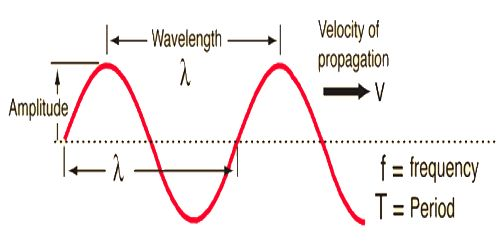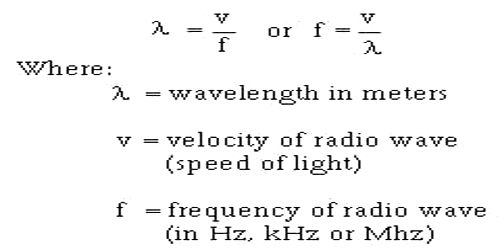Physics

# Relation among Wavelength, Frequency and Wave VelocityRelation among wavelength, frequency and wave velocity

Let the velocity of the wave in a medium be v, frequency of the source of the wave n and wavelength λ. A relation is to be found out among these quantities.

The wavelength and frequency of light are intimately connected. The higher the frequency, the shorter the wavelength. Because all light waves shift all the way through a vacuum at the similar speed, the number of wave crests passing by a given point in one second depends on the wavelength.

Since the frequency of the wave is n, so, n numbers of waves are produced in one second and the particles of the medium complete n vibrations. Now after one complete vibration of the particle, the wave travels a distance λ. So after f vibrations, the wave travels a distance fλ. This distance is travelled in one second which is the velocity of the wave.

v = fλ

i.e., velocity = frequency x wavelength.This is the relation among velocity, frequency and wavelength of a wave. This is a general wave relationship which applies to sound and light waves, other electromagnetic waves, and waves in mechanical media.

For any given medium, wavelength and frequency are directly proportional (longer wavelength = slower frequency). Wave velocity is highly dependent upon the medium through which the wave is propagating.